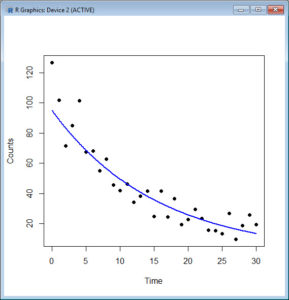In Parts 3 and 4 we used the `lm()` command to perform least squares regressions. We saw how to check for non-linearity in our data by fitting polynomial models and checking whether they fit the data better than a linear model. Now let’s see how to fit an exponential model in R.

As before, we will use a dataset of counts (atomic disintegration events that take place within a radiation source), taken with a Geiger counter at a nuclear plant. The counts were registered over a 30 second period for a short-lived, man-made radioactive compound. We read in the data and subtract the background count of 623.4 counts per second in order to obtain the counts that pertain to the radio-active source. As before, we cut and paste the following data into our R workspace.

```geiger <- structure(list(Time = c(0, 1, 2, 3, 4, 5, 6, 7, 8, 9, 10, 11, 12, 13, 14, 15, 16, 17, 18, 19, 20, 21, 22, 23, 24, 25, 26, 27, 28, 29, 30), Counts = c(750, 725.2, 695, 708.5, 725, 690.9, 691.5, 678.6, 686.3, 668.9, 665.3, 669.7, 657.5, 661.6, 665.1, 648.1, 664.9, 647.9, 660, 643, 646.2, 653, 646.9, 639.3, 638.7, 636.8, 650.2, 633.2, 642.2, 649.3, 642.7)), .Names = c("Time", "Counts"), row.names = c(NA, -31L), class = "data.frame") geiger```

Again, we subtract the background count from the data set.

```backgroundcount <- 623.4 geiger\$Counts <- geiger\$Counts - backgroundcount```

Let’s attach the entire dataset so that we can refer to all variables directly by name.

```attach(geiger) names(geiger)```

Since the physics of atomic decay involves exponential relationships, let’s see if an exponential function fits the data even better than a quadratic. An exponential function in the `Time` variable can be treated as a model of the log of the `Counts` variable.

```exponential.model <- lm(log(Counts)~ Time) summary(exponential.model)```

R returns the following output:This model is pretty good, though it explains about 83% of the variance by comparison with the 90% explained by the quadratic model. Let’s plot it on a grid of time values from 0 to 30 in intervals of 0.1 seconds.

```timevalues <- seq(0, 30, 0.1) Counts.exponential2 <- exp(predict(exponential.model,list(Time=timevalues))) plot(Time, Counts,pch=16) lines(timevalues, Counts.exponential2, lwd=2, col = "blue", xlab = "Time (s)", ylab = "Counts per Second")```

Note that we used the exponential of the predicted values.So – we have fitted our exponential model. Although the physics of atomic decay is exponential, in this case the fitted exponential model fits the data less well than the quadratic model.

That’s all for now. Next time we will look at some basic plotting syntax.

David

#### Annex: R codes used

```# Inserting Geiger dataset into R workspace
geiger <- structure(list(Time = c(0, 1, 2, 3, 4, 5, 6, 7, 8, 9, 10, 11, 12, 13, 14, 15, 16, 17, 18, 19, 20, 21, 22, 23, 24, 25, 26, 27, 28, 29, 30), Counts = c(750, 725.2, 695, 708.5, 725, 690.9, 691.5, 678.6, 686.3, 668.9, 665.3, 669.7, 657.5, 661.6, 665.1, 648.1, 664.9, 647.9, 660, 643, 646.2, 653, 646.9, 639.3, 638.7, 636.8, 650.2, 633.2, 642.2, 649.3, 642.7)), .Names = c("Time", "Counts"), row.names = c(NA, -31L), class = "data.frame")
geiger

# Obtaining counts that pertain to the radio-active
# source by subtracting the background count
backgroundcount <- 623.4
geiger\$Counts <- geiger\$Counts - backgroundcount

# Attaching dataset and referring variables by names
attach(geiger)
names(geiger)

# Estimating exponential regression model
exponential.model <- lm(log(Counts)~ Time)
summary(exponential.model)

# Scatterplot and exponential regression model
timevalues <- seq(0, 30, 0.1)
Counts.exponential2 <- exp(predict(exponential.model, list(Time=timevalues)))
plot(Time, Counts,pch=16)
lines(timevalues, Counts.exponential2, lwd=2, col = "blue", xlab = "Time (s)", ylab = "Counts per Second")
```